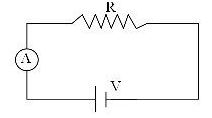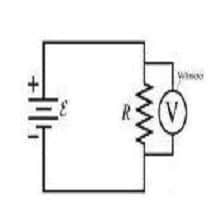Physic Tutorials

# Why Ammeter is Connected in Series And Voltmeter connected in Parallel

Before I explain why ammeter is connected in series and voltmeter is connected in parallel in a circuit, there is a need to explain the function of the two measuring instruments. This will help you to understand what I am about to explain.

Function of Ammeter

It is used to measure the value of flowing current in a circuit.

Function of Voltmeter

It is used to measure the value of the potential difference or voltage across the load (resistor). The reading on the voltmeter indicates the energy transferred to the component by each unit of the charge.

Now you need to understand series and parallel connection;

In series connection,

• Current are the same through the loads
• In a closed circuit only ONE path for current to flow
• Total potential difference = sum of individual potential difference across the loads
• Total resistance = sum of individual resistances

In parallel connection,

• Potential difference across each resistor
• Total current = sum of individual currents
• Effective resistance less than individual resistance

## Why ammeter is connected in series

The ammeter is connected in series with the load so that there is the same current in both. This can only be possible if the resistance on the ammeter is very low so as to ensure correct measurement of current in the circuit.For an ideal ammeter, the value of the resistance should be zero. This means that all the voltage should appear across the load, so that current can be measured accurately.

From, V =IR

Voltage is directly proportional to current flow. If there is no voltage on the ammeter, then current won’t flow. And this can only be possible if the resistance on the ammeter is zero.

## Why voltmeter is connected in parallel

A voltmeter is designed to have a high resistance and should be connected in parallel in order to measure accurately.

By this, no current will flow through the voltmeter i.e. it won’t disrupt the current that should flow across a resistor or load.For current not to flow through a voltmeter, the resistance of an ideal voltmeter should be infinity.

Kindly note current in a circuit will choose to flow through a less resistive path. This is also a reason why the resistance of a voltmeter must be high to avoid current flow across it.

Mathematically,

If the load is r and the voltmeter resistance is infinity connected in parallel to each other, the effective resistance will be

1/r + 1/infinity = 1/R

1/infinity = 0

1/r + 0 = 1/R

R = r (i.e. the effective resistance is the same as the resistance on the load)

This shows clearly that volt-meter will not alter the flow of current in the circuit.

Recommended: Note on Direct Current

### Bolarinwa Olajire

A lecturer, Educationist, PhD student at FUNAAB, and a Blogger.